Mathematics
Easy

Question

#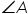is called _________.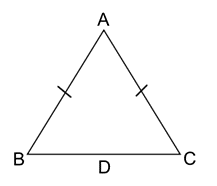## Base AngleLegHypotenuseVertex AngleHint:

## The correct answer is: Vertex Angle

Mathematics

Mathematics

Mathematics

### Can the HL congruence theorem be used to prove the triangles congruent? If so, write a congruence statement.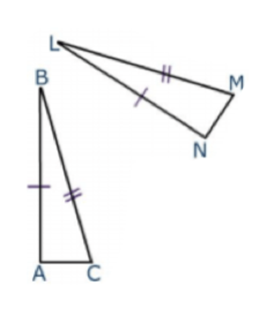Mathematics

Mathematics

Mathematics

### If ∆ ABC and ∆ PQR are to be congruent, then name one additional pair of corresponding parts.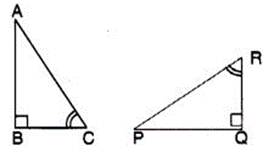Mathematics

### Complete the congruence statement: ∆ BCA =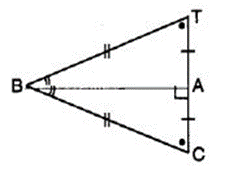Congruent triangles are triangles that have same size and shape. This suggests us that the corresponding sides are equal and corresponding angles are equal.
When two triangles are congruent, all of their corresponding sides and angles are equal.

### Complete the congruence statement: ∆ BCA =Congruent triangles are triangles that have same size and shape. This suggests us that the corresponding sides are equal and corresponding angles are equal.
When two triangles are congruent, all of their corresponding sides and angles are equal.

Mathematics

### We want to show that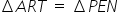. We have to use SAS criterion. We have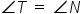, RT = EN, then____________.

SAS congruence is the term which is also known as Side Angle Side congruence. In this, triangles are congruent if any pair of corresponding sides and their included angles are equal in both triangles.

### We want to show that. We have to use SAS criterion. We have, RT = EN, then____________.

SAS congruence is the term which is also known as Side Angle Side congruence. In this, triangles are congruent if any pair of corresponding sides and their included angles are equal in both triangles.

Mathematics

### For two given triangles, ΔABC and ΔPQR, the number of possible matchings are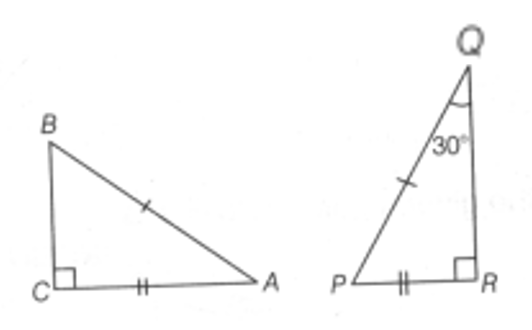Mathematics

Mathematics

### ∆ PQR is congruent to ∆ STU (in figure), then length of TU is ___________.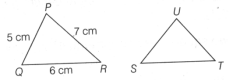Congruent triangles are triangles that have same size and shape. This suggests us that the corresponding sides are equal and corresponding angles are equal.
When two triangles are congruent, all of their corresponding sides and angles are equal.

### ∆ PQR is congruent to ∆ STU (in figure), then length of TU is ___________.Congruent triangles are triangles that have same size and shape. This suggests us that the corresponding sides are equal and corresponding angles are equal.
When two triangles are congruent, all of their corresponding sides and angles are equal.

Mathematics

### If, for ∆ ABC and ∆ DEF, the correspondence CAB and EDF gives a congruence, then false statement isMathematics

Mathematics

### The symbol of correspondence is

The double-sided arrow symbol is used to denote correspondence

### The symbol of correspondence is

The double-sided arrow symbol is used to denote correspondence

Mathematics

### In the given figure, if ,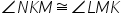then ___________.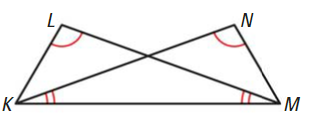#### With Turito Foundation.#### Get an Expert Advice From Turito.